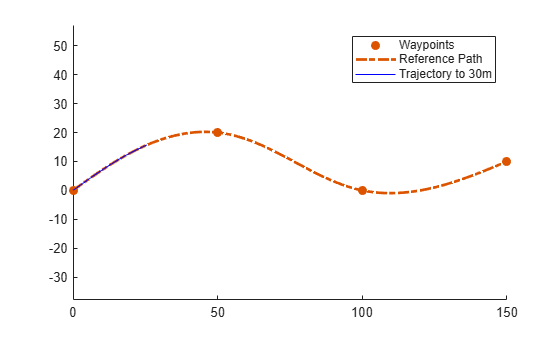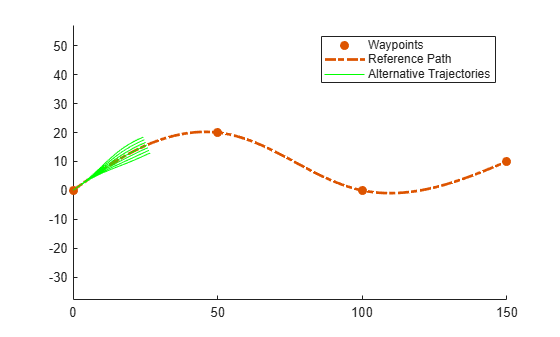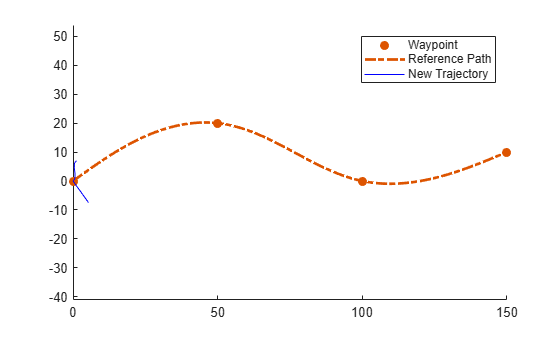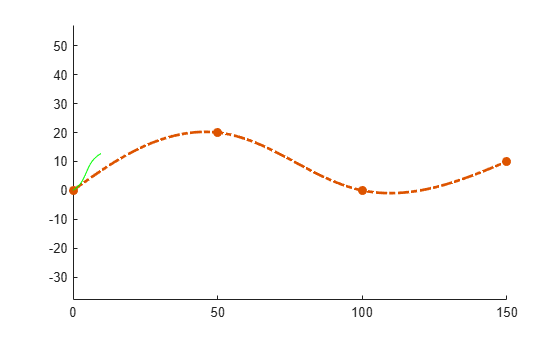# show

Display reference path in figure

Since R2020b

## Syntax

``show(refPath)``
``show(refPath,'Parent',parentAx)``
``ax = show(___)``

## Description

example

````show(refPath)` displays the reference path and its lateral states in the current figure.```
````show(refPath,'Parent',parentAx)` displays the reference path on the specified axes. `parentAx` is specified as an `Axes` handle.```
````ax = show(___)` displays the reference path using any of the previous input combinations and returns the axes handle on which the reference path is plotted.```

## Examples

collapse all

Generate alternative trajectories for a reference path using Frenet coordinates. Specify different initial and terminal states for your trajectories. Tune your states based on the generated trajectories.

Generate a reference path from a set of waypoints. Create a `trajectoryGeneratorFrenet` object from the reference path.

```waypoints = [0 0; ... 50 20; ... 100 0; ... 150 10]; refPath = referencePathFrenet(waypoints); connector = trajectoryGeneratorFrenet(refPath);```

Generate a five-second trajectory between the path origin and a point 30 m down the path as Frenet states.

```initState = [0 0 0 0 0 0]; % [S ds ddS L dL ddL] termState = [30 0 0 0 0 0]; % [S ds ddS L dL ddL] [~,trajGlobal] = connect(connector,initState,termState,5);```

Display the trajectory in global coordinates.

```show(refPath); hold on axis equal plot(trajGlobal.Trajectory(:,1),trajGlobal.Trajectory(:,2),'b') legend(["Waypoints","Reference Path","Trajectory to 30m"])```Create a matrix of terminal states with lateral deviations between –3 m and 3 m. Generate trajectories that cover the same arc length in 10 seconds, but deviate laterally from the reference path. Display the new alternative paths.

```termStateDeviated = termState + ([-3:3]' * [0 0 0 1 0 0]); [~,trajGlobal] = connect(connector,initState,termStateDeviated,10); clf show(refPath); hold on axis equal for i = 1:length(trajGlobal) plot(trajGlobal(i).Trajectory(:,1),trajGlobal(i).Trajectory(:,2),'g') end legend(["Waypoints","Reference Path","Alternative Trajectories"]) hold off```Specify a new terminal state to generate a new trajectory. This trajectory is not desirable because it requires reverse motion to achieve a lateral velocity of 10 m/s.

```newTermState = [5 10 0 5 0 0]; [~,newTrajGlobal] = connect(connector,initState,newTermState,3); clf show(refPath); hold on axis equal plot(newTrajGlobal.Trajectory(:,1),newTrajGlobal.Trajectory(:,2),'b'); legend(["Waypoint","Reference Path","New Trajectory"]) hold off```Relax the restriction on the longitudinal state by specifying an arc length of `NaN`. Generate and display the trajectory again. The new position shows a good alternative trajectory that deviates off the reference path.

```relaxedTermState = [NaN 10 0 5 0 0]; [~,trajGlobalRelaxed] = connect(connector,initState,relaxedTermState,3); clf show(refPath); hold on axis equal plot(trajGlobalRelaxed.Trajectory(:,1),trajGlobalRelaxed.Trajectory(:,2),'g'); hold off```## Input Arguments

collapse all

Reference path, specified as a `referencePathFrenet` object.

## Output Arguments

collapse all

Axes on which the reference path is plotted, returned as an `Axes` handle.

## Version History

Introduced in R2020b